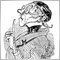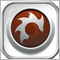# EA won´ place SL/TP11

Hi, I have a problem with my EA. When I run tester in MT4, EA place TP/SL properly TP (100 pips) and SL ( 50pips) but when i try to run my EA on demo acc with same setting, it won´t open a possition due to the small TP/SL I think( if I made an order manually and place manually TP/SL with same settings, it is no problem). So here I thought that instead of placing order with TP/SL at the same time, my EA will place an order and after that, it will place TP/SL. It is an ECN broker. Sorry for my english.

Here is the code:

```extern int MagicNumber=10001;
extern double Lots =0.1;
extern double StopLoss=50;
extern double TakeProfit=100;
extern int TrailingStop=50;
extern int Slippage=3;
//+------------------------------------------------------------------+
//    expert start function
//+------------------------------------------------------------------+
int start()
{
double MyPoint=Point;
if(Digits==3 || Digits==5) MyPoint=Point*10;

double TheStopLoss=0;
double TheTakeProfit=0;
if( TotalOrdersCount()==0 )
{
int result=0;
{
if(result>0)
{
TheStopLoss=0;
TheTakeProfit=0;
OrderSelect(result,SELECT_BY_TICKET);
OrderModify(OrderTicket(),OrderOpenPrice(),NormalizeDouble(TheStopLoss,Digits),NormalizeDouble(TheTakeProfit,Digits),0,Green);
}
return(0);
}
if((Close[-1]<iMA(NULL,0,34,0,MODE_EMA,PRICE_CLOSE,0))&&(Close[-1]<iMA(NULL,0,34,0,MODE_EMA,PRICE_OPEN,0))&&(Close[-1]<iMA(NULL,0,34,0,MODE_EMA,PRICE_HIGH,0))&&(Close[-1]<iMA(NULL,0,34,0,MODE_EMA,PRICE_LOW,0))) // Here is your open Sell rule
{
if(result>0)
{
TheStopLoss=0;
TheTakeProfit=0;
if(TakeProfit>0) TheTakeProfit=Bid-TakeProfit*MyPoint;
if(StopLoss>0) TheStopLoss=Bid+StopLoss*MyPoint;
OrderSelect(result,SELECT_BY_TICKET);
OrderModify(OrderTicket(),OrderOpenPrice(),NormalizeDouble(TheStopLoss,Digits),NormalizeDouble(TheTakeProfit,Digits),0,Green);
}
return(0);
}
}

for(int cnt=0;cnt<OrdersTotal();cnt++)
{
if(OrderType()<=OP_SELL &&
OrderSymbol()==Symbol() &&
OrderMagicNumber()==MagicNumber
)
{
{
if(TrailingStop>0)
{
if(Bid-OrderOpenPrice()>MyPoint*TrailingStop)
{
if(OrderStopLoss()<Bid-MyPoint*TrailingStop)
{
OrderModify(OrderTicket(),OrderOpenPrice(),Bid-TrailingStop*MyPoint,OrderTakeProfit(),0,Green);
return(0);
}
}
}
}
else
{
if(TrailingStop>0)
{
{
{
return(0);
}
}
}
}
}
}
return(0);
}

int TotalOrdersCount()
{
int result=0;
for(int i=0;i<OrdersTotal();i++)
{
if (OrderMagicNumber()==MagicNumber) result++;

}
return (result);
}
{
int i;
bool profit1=false;
int SystemHistoryOrders=0;
for( i=0;i<OrdersHistoryTotal();i++)
{  OrderSelect(i,SELECT_BY_POS ,MODE_HISTORY);
if (OrderMagicNumber()==MagicNumber) SystemHistoryOrders++;
}
bool profit2=false;
int LO=0;
if(SystemHistoryOrders<2) return(Lots);
for( i=OrdersHistoryTotal()-1;i>=0;i--)
{
if(OrderSelect(i,SELECT_BY_POS ,MODE_HISTORY))
if (OrderMagicNumber()==MagicNumber)
{
if(OrderProfit()>=0 && profit1) return(Lots);
if( LO==0)
{  if(OrderProfit()>=0) profit1=true;
if(OrderProfit()<0)  return(OrderLots());
LO=1;
}
if(OrderProfit()>=0) profit2=true;
if(OrderProfit()<0 )
{   profit1=false;
profit2=false;
}
}
}
}```Moderator
12283

2) and reduce the wideness of your lines!Moderator
12283

To see one line totally I have to reduce the font-size so much that I can't read any more what you have written. :(Moderator
32153

gooly:Check your return codes (OrderSelect and OrderModify) What are Function return values ? How do I use them ? - MQL4 forum and Common Errors in MQL4 Programs and How to Avoid Them - MQL4 Articles ```  for(int cnt=0;cnt= 0; --cnt) if(      OrderSelect(cnt, SELECT_BY_POS)   && OrderType()        <= OP_SELL   && OrderMagicNumber() == MagicNumber   && OrderSymbol()      == Symbol()   ){``` Are your books one column but two feet wide? No because that is unreadable. They are 6 inches, sometimes two columns, so you can read it easily. So should be your code. I'm not going to go scrolling back and forth trying to read it. Edit the post with formatted code and you might get additional help.Do NOT use NormalizeDouble, EVER. For ANY Reason. It's a kludge, don't use it. It's use is always wrong SL/TP are market orders when triggered, they don't need to be normalized, only abide by the limits Requirements and Limitations in Making Trades - Appendixes - MQL4 Tutorial and that requires understanding floating point equality Can price != price ? - MQL4 forum Only the open price for pending orders need to be adjusted. On Currencies, Point == TickSize, so you will get the same answer, but it won't work on Metals. So do it right: Trailing Bar Entry EA - MQL4 forum or Bid/Ask: (No Need) to use NormalizeDouble in OrderSend - MQL4 forum Lot size must also be adjusted to a multiple of LotStep. If that is not a power of 1/10 then NormalizeDouble is wrong. Do it right. OrderModify(OrderTicket(), OrderOpenPrice(), NormalizeDouble(TheStopLoss,Digits), NormalizeDouble(TheTakeProfit,Digits), 0, Green); Use self-documenting variable names. MyPoint converts PIPs to price. Shouldn't be called point.You convert PIPs to prices fine but you don't convert Slippage from PIPs to points.See pips2dbl ```  double MyPoint=Point;   if(Digits==3 || Digits==5) MyPoint=Point*10; ``` Current, forming bar is zero. Previous bar is 1. The unknown future is -1 ` if((Close[-1]